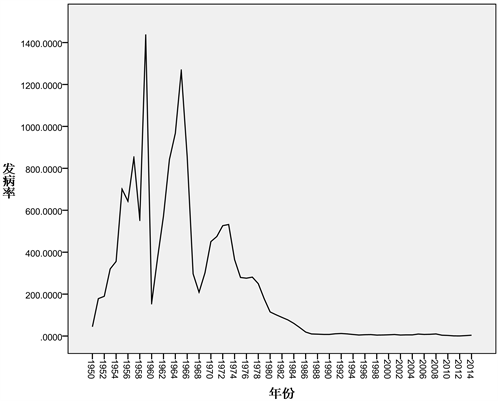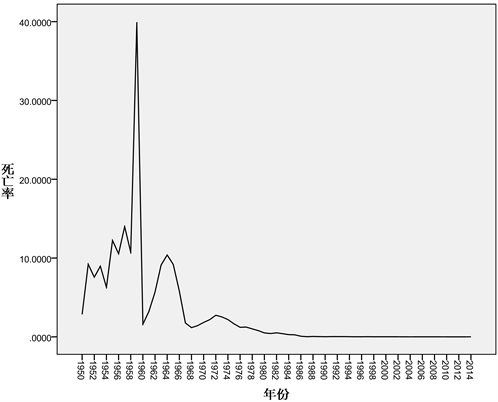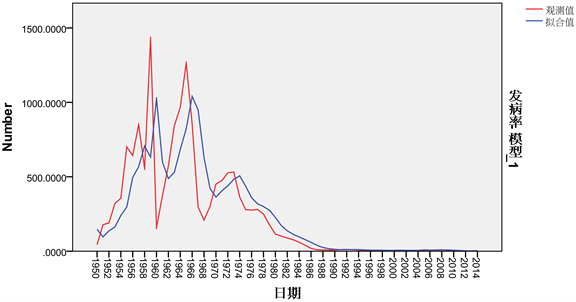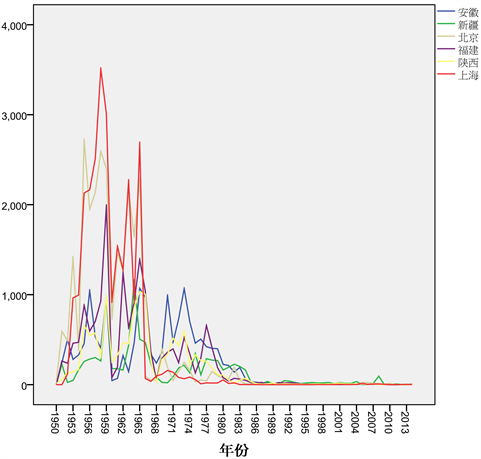# 基于时间序列的麻疹发病率分析Analysis of Measles Incidence Based on Time Series

DOI: 10.12677/SA.2017.65062, PDF, HTML, XML, 下载: 792  浏览: 1,984  科研立项经费支持

Abstract: Based on the national measles morbidity data from 1950 to 2014, we establish a model to effec-tively predict the incidence of measles in the short term through the time series analysis method, so as to issue warnings on the epidemic situation of measles and prepare the preventive work in advance.

1. 引言

2. 主要内容

2.1. 背景

2.2. 数据来源Figure 1. Morbidity time series chartFigure 2. Mortality time series chart

2.3. 模型

20世纪60年代，George Box与Gwilym Jenkins提出了一种关于时间序列分析、预测的方法，称之为B-J模型，也叫做ARMA (Auto Regression Moving Average)模型。ARMA模型  的基本模型有三种情况：自回归模型(AR模型)；滑动平均模型(MA模型)；自回归滑动平均模型(ARMA模型)  。

${\stackrel{^}{X}}_{t+1}=\alpha {X}_{t}+\alpha \left(1-\alpha \right){\stackrel{^}{X}}_{t}$

${\stackrel{^}{X}}_{t+1}=\alpha {X}_{t}+\alpha \left(1-\alpha \right){X}_{t-1}+\alpha {\left(1-\alpha \right)}^{2}{X}_{t-2}+\cdots +\alpha {\left(1-\alpha \right)}^{t-1}{X}_{1}+{\left(1-\alpha \right)}^{t}{\stackrel{^}{X}}_{1}$

2.4. 结论Figure 3. Observation chartsFigure 4. Measles incidence map around

  Glass, K., Xia, Y. and Grenfell, B.T. (2003) Interpreting Time-Series Analyses for Continuous-Time Biological Models—Measles as a Case Study. Journal of Theoretical Biology, 223, 19-25. https://doi.org/10.1016/S0022-5193(03)00031-6  田鑫, 陈超, 等. 应用时间序列模型预测麻疹疫情[J]. 中国疫苗和免疫, 2008, 14(3): 229-233.  刘曙光, 王立芹, 等. 河北省麻疹疫情时间序列的预测和预警分析[J]. 中国卫生检验杂志, 2015, 25(17): 2954-2956.  丁晓艳, 彭志行, 等. 运用时间序列模型对麻疹流行趋势的预测与分析[J]. 南京医科大学学报, 2011, 31(8): 1200-1203.  姜乐. 基于时间序列的股票价格分析研究与应用[D]: [硕士学位论文]. 大连: 大连理工大学, 2015.  郑彦玲, 秦伶俐, 张利萍. 基于随机时间序列法的新疆麻疹发病率预测[J]. 数学的实践与认识, 2012, 16(42): 178-184.  骆方, 刘红云. SPSS数据统计与分析[M]. 北京: 清华大学出版社, 2011.  刘曙光, 王立芹, 等. 河北省流行性腮腺炎SARIMA模型预测分析[J]. 医学动物防制, 2015, 31(9): 968-970.